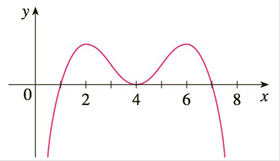Chapter 3.3, Problem 7E

Chapter
Section
Textbook Problem

# In each part state the x-coordinates of the inflection points of f. Give reasons for your answers.(a) The curve is the graph of f.(b) The curve is the graph of f'.(c) The curve is the graph of f''.To determine

(a)

To find:

The x coordinates of the inflection points if the curve is graph of f.

Explanation

1) Concept:

The points where the concavity changes from upward to downward or downward to upward are inflection points.

2) Calculation:

The graph changes from concave downward to concave upward at x=3.

It changes from concave upward to concave downward at x =

To determine

(b)

To find:

The x coordinates of the inflection points if the curve is graph of f'.

To determine

(c)

To find:

The x coordinates of the inflection points if the curve is graph of f''.

### Still sussing out bartleby?

Check out a sample textbook solution.

See a sample solution

#### The Solution to Your Study Problems

Bartleby provides explanations to thousands of textbook problems written by our experts, many with advanced degrees!

Get Started